# Complete set

(diff) ← Older revision | Latest revision (diff) | Newer revision → (diff)

in a topological vector spaceover a fieldA setsuch that the set of linear combinations of the elements fromis (everywhere) dense in, i.e. the closed subspace generated by the set, i.e. the closed linear hull of, coincides with. For example, in the normed spaceof continuous functions on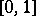with values inthe set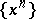is a complete set. Ifis a non-discretely normed field, each absorbing set (and in particular each neighbourhood of zero in) is a complete set.

In order for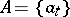,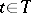, to be a complete set in the weak topology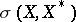of a spaceit is necessary and sufficient that there exist an indexsuch that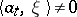for each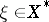; this means that no closed hyperplane contains all the elements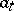, i.e. thatis a total set. Moreover, ifis a locally convex space, a complete set in the weak topology will be a complete set in the initial topology also.

Of course, a complete set in a topological vector space can also mean a setsuch that every Cauchy sequence inconverges in; and this is by far the most frequently occurring meaning of the phrase. For the notion of an absorbing set cf. Topological vector space.